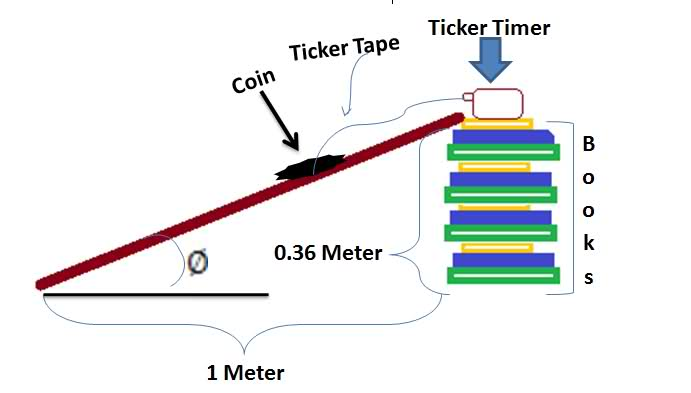# Kinetic Friction

## Homework Statement

I have a lab on friction I did in class, I have missed couple of days and and its due today, what i need to know is how can i calculate the friction of the object if I have the following given , the time it took for the object to come down the raamp, the displacement , the height of the inclined surface and length.

Note I want to know the coefficient kinetic friction, not static.( i know how i can get that)

## Homework Equations

I don't really know which one to go with.

## The Attempt at a Solution## Answers and Replies

rock.freak667
Homework Helper
Using s=ut+0.5at2 you can get the resultant acceleration of the car.

If you apply Newton's 2nd Law to the car, what expression do you get?